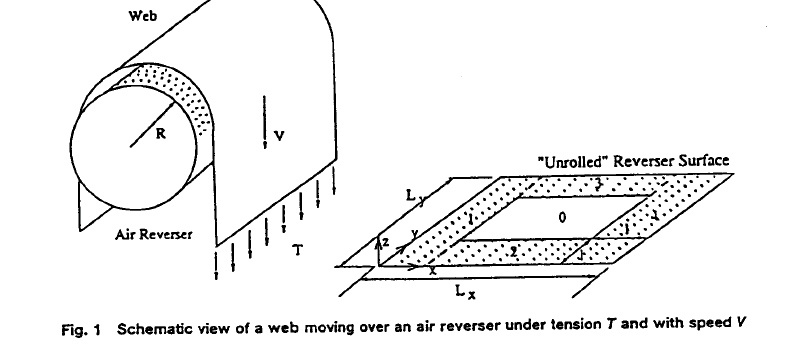Industrial Applications... from Concept to Completion

Industrial Applications...
from Concept to Completion

Looking for a Numerical Solution of the Euler's Equations with Nonlinear Source Terms?

Look no further and download this paper! Here we report on the general equations governing the 2D air flow in the web-reverser gap assuming that the location of the web is fixed and known.  In this paper we:• A two-dimensional model of the air flow obtained by averaging the equations of conservation of mass and mommentum over the clearance between the web and the reverser is solved numerically.
• The effect of the pressure holes in the surface of the air reverser are represented by nonlinear source terms in the modified Euler's and mass conservation equations
• The results of the numerical solution are compared with a 1D analytical solution of these equations, and empirical formula and the one-dimensional air-jet theory developed for hovercraft.
• Conditions that maximize the air pressure supporting the web are analyzed and design guideline deduced.